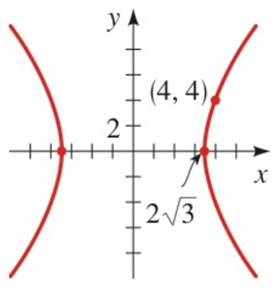Chapter 12.3, Problem 30E### Algebra and Trigonometry (MindTap ...

4th Edition
James Stewart + 2 others
ISBN: 9781305071742

#### Solutions

Chapter
Section### Algebra and Trigonometry (MindTap ...

4th Edition
James Stewart + 2 others
ISBN: 9781305071742
Textbook Problem

# 2 7 - 3 2 . Finding an equation of a Hyperbola Find the equation of hyperbola whose graph is shown.To determine

To find:

The equation of a hyperbola.

Explanation

Given:

Figure (1)

Approach:

The equation of a hyperbola with a horizontal transverse axis is as follows.

x2a2y2b2=1,a>0,b>0...(1)

The vertices are (±a,0).

Calculation:

From the graph the vertices are (±23,0). Then,

a=23

Since the point (4,4) lies on the given hyperbola so it should satisfy (1).

Substitute 23 for a, and (4,4) for (x,y) in the equation (1)

### Still sussing out bartleby?

Check out a sample textbook solution.

See a sample solution

#### The Solution to Your Study Problems

Bartleby provides explanations to thousands of textbook problems written by our experts, many with advanced degrees!

Get Started